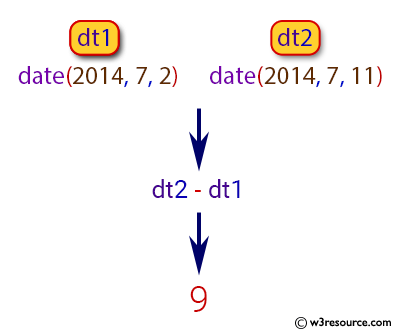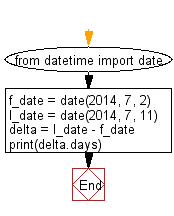﻿ Python: Calculate number of days between two dates - w3resource# Python: Calculate number of days between two dates

## Python Basic: Exercise-14 with Solution

Write a Python program to calculate number of days between two dates.

Python datetime.date(year, month, day) :

The function returns date object with same year, month and day. All arguments are required. Arguments may be integers, in the following ranges:

• MINYEAR <= year <= MAXYEAR
• 1 <= month <= 12
• 1 <= day <= number of days in the given month and year

If an argument outside those ranges is given, ValueError is raised.

• Note: The smallest year number allowed in a date or datetime object. MINYEAR is 1.
• The largest year number allowed in a date or datetime object. MAXYEAR is 9999.

Pictorial Presentation:Sample Solution:-

Python Code:

``````from datetime import date
f_date = date(2014, 7, 2)
l_date = date(2014, 7, 11)
delta = l_date - f_date
print(delta.days)
```
```

Sample Output:

```9
```

Flowchart:## Visualize Python code execution:

The following tool visualize what the computer is doing step-by-step as it executes the said program:

Python Code Editor:

Have another way to solve this solution? Contribute your code (and comments) through Disqus.

What is the difficulty level of this exercise?

Test your Python skills with w3resource's quiz

﻿

## Python: Tips of the Day

Unknown Arguments Using *arguments:

If your function can take in any number of arguments then add a * in front of the parameter name:

```def myfunc(*arguments):
for a in arguments:
print a
myfunc(a)
myfunc(a,b)
myfunc(a,b,c)
```